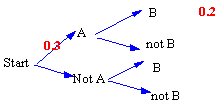### CFA Practice Question

There are 985 practice questions for this topic.

### CFA Practice Question

For the tree diagram shown below:P(not B | A) = ______

A. 0.1
B. 2/3
C. 1/3

From the tree diagram, we know that 0.3 times P(B | A) = 0.2. Now, 0.3x = 0.2 yields x = 0.2/0.3 = 2/3. Thus, P(B | A) = 2/3. We have: P(B | A) + P(not B | A) = 1. Finally, 2/3 + P(not B | A) = 1 and P(not B | A) = 1 - 2/3 = 1/3.

User Comment
stevelaz Can someone explain this?
BayAreaPablo I agree how can P(B|A) go from .2 to .67?
BayAreaPablo I get it:
P(B|A)x P(A)=P(AB)
therefore,
P(B|A)=?
P(A)=.3
P(AB)=.2
Therefore:
P(B|A)x.3=.2
P(B|A)=2/3
Now that you have this:Remember
P(B|A) + P(not B|A)=1
Now solve for P(not B|A)
surob I don't still understand why 0.2 = P(AB)?
cp24 What's misleading is that probability 0.2 is not P(B/A) but P(BA).
epizi I don't think there is any problem,because the tree indicates that A happens before B, not both happening same time.Therefore P(A)=0.3 and P(B)=?
But the P(AB)=0.2
P(AB)=P(A)xP(B/A)
0.2=0.3xP(B/A)
P(B/A)=2/3
P(B/A) and P(notB/A) are exhaustive ie sum up to 1

So 1-P(B/A)=P(not B/A)
Hence 1-2/3= 1/3

Hope this makes it clear, I did not get it from first try either
StanleyMo thanks epizi. nice explain.
JKiro I find this a bit more clearer...
what we know:
p(A) * p(B|A) = 0.2; we also know p(A) = 0.3
solving for p(B|A): 0.2/0.3 = 0.6667
what we also know:
p(B|A) + p(not B|A) must equal 1
we have calculated p(B|A)
solving for p(not B|A): 1 - 0.6667 = 0.3333
fedha JKiro I am not sure how you know p(B|A)is 0.2??
I hope you meant p(BA)= 0.2

Here is how would solve this problem. Epizi is right in solving the problem

P(B|A) = P(BA)/P(A)
P(B|A) = 0.2/0.3
P((B|A) = 0.667
We know that P(B|A) + P(Not B|A) = 1
We already solved for P(B|A) = 0.0667 Therefore
P(Not B|A) = 1-0.667 = 0.333 ~ 1/3
loisliu88 I don't understand how can P(B/A)+P(notB/A)=1, what about P(notA). shouldn't it be P(B/A)+P(notB/A)+P(notA/B)+P(notA/notB)=1? plz explain it to me.
harpalani Good explanation epizi!!
LordRommel Oh. It is simple. 0.01/0.03 = 1/3
Saxonomy If I understood the diagram, the answer would be easy.

Loisliu88, too much going on in your comment:
P(B|A) + P(notB|A) = 1
Ignore the "|A", just know that B + "notB" will always be 1.

Prob that LeBron wins the title (given the fact that the Lakers/Celtics are out) + the prob that LeBron does not win the title (given the fact that the Lakers/Celtics are out) equals 1.
2014 Good explnation Fedha
sgossett86 CFAs, this problem upset me. Like they say above, turns out I wasn't reading the chart right.

.2=P(AB)
.3=P(A)

P(A|B)=P(AB)/P(A)=.2/.3

P(A|B)+P(A|notB)=1

.2/.3+P(A|notB)=1

P(A|notB)=1/3
Scc0813 Why is 0.2 P(AB) and not P(B|A)?
Safiya921 I didn't read the Tree Diagram correct. I thought P(B I A) = 0.2, then I got to know from your comments that it's P (AB) = 0.2.
Thank you all.